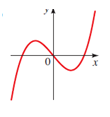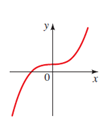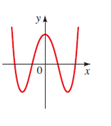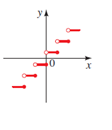# the given function in the figure is even, odd or neither.### Precalculus: Mathematics for Calcu...

6th Edition
Stewart + 5 others
Publisher: Cengage Learning
ISBN: 9780840068071### Precalculus: Mathematics for Calcu...

6th Edition
Stewart + 5 others
Publisher: Cengage Learning
ISBN: 9780840068071

#### Solutions

Chapter 2, Problem 66RE

a.

To determine

## To determine the given function in the figure is even, odd or neither.

Expert Solution

The given function for this graph is odd.

### Explanation of Solution

Given figure:As the graph is symmetric with respect to the origin. So, function for this graph is odd.

b.

To determine

### To determine the given function in the figure is even, odd or neither.

Expert Solution

The given function is neither symmetric with y-axis, nor symmetric with origin.

### Explanation of Solution

The given figure:Observe the above graph; this is neither symmetric with y-axis, nor symmetric with origin.

So, this graph is even, nor odd.

c.

To determine

### To determine the given function in the figure is even, odd or neither.

Expert Solution

The given figure is symmetric with y-axis.so it must be an even function graph.

### Explanation of Solution

The given figure:Observe the above graph, it is symmetric with y-axis.so it must be an even function graph.

d.

To determine

### To determine the given function in the figure is even, odd or neither.

Expert Solution

The given figure is symmetric with origin. So, this is an odd function.

### Explanation of Solution

The given figure:Observe the above graph, this is graph is symmetric with the origin. So, it is symmetric with origin.

So, this is an odd function.

### Have a homework question?

Subscribe to bartleby learn! Ask subject matter experts 30 homework questions each month. Plus, you’ll have access to millions of step-by-step textbook answers!